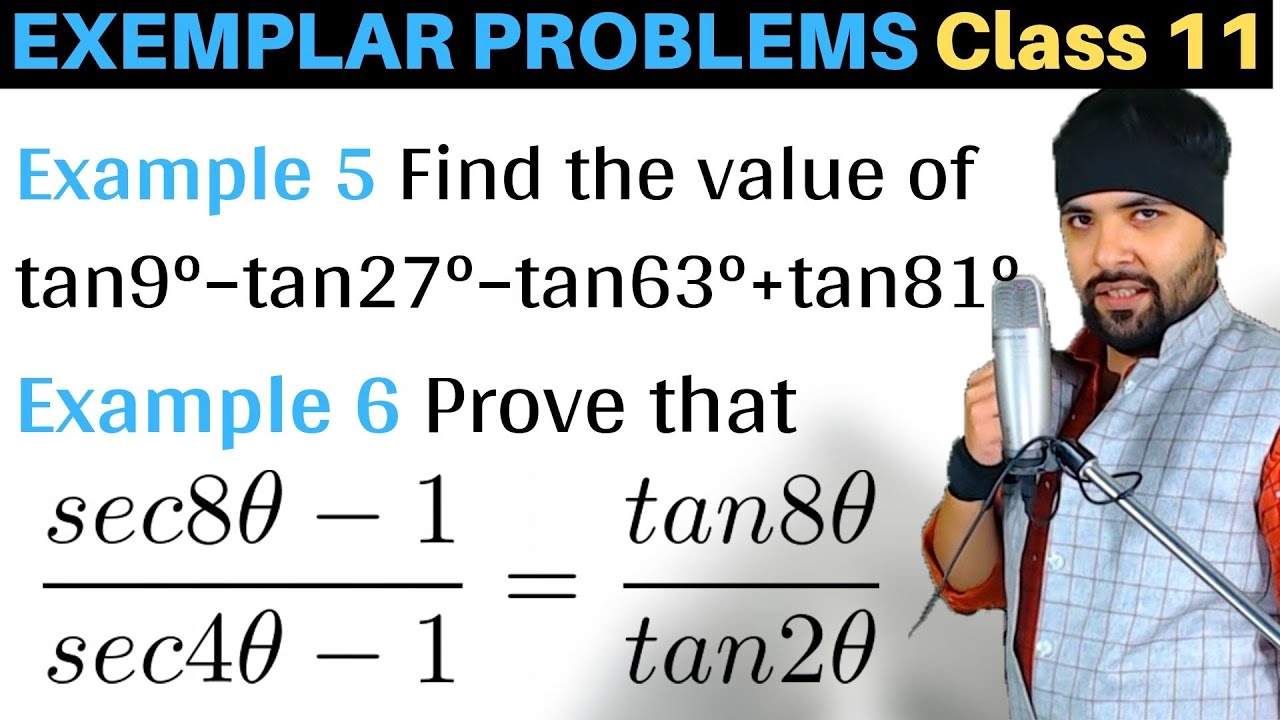# Prove that (sec8θ−1)/(sec4θ−1)=tan8θ/tan2θ## Topics/Questions discussed in this video:

NCERT Exemplar Class 11 Maths Chapter 3 Trigonometry Functions

Example 6: Prove that \frac{sec8\theta-1}{sec4\theta-1}=\frac{tan8\theta}{tan2\theta}